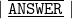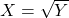Question

what is the formula of finding sqare rood?​

1.minhkhangThere’s no specific formula to find the square root of a number, however we can find the square root of a number by the following methods:

i) By Prime Factorisation

ii) By Long Division

iii) By Repeated subtraction method

## ✏ By Prime Factorisation:

Step I: Obtain the given number.

Step II: Reduce the given number into prime factors by successive division.

Step III: Now make pairs of prime factors in such a way that both the factors in each pair are equal.

Step IV: Take one factor from each pair and find the product of these factors.

Step V: The product obtained by multiplying the factors is the required square root.

## ✏ Long Division:

Step 1: Firstly, we place a bar on every pair of digits starting from the unit digit. If the number of digits in it is odd, we put a bar on the single-digit too.

Step 2: Now we find the largest number whose square is less than or equal to the 1st number.

Step 3: Now we bring down the next bar number.

Step 4: For new divisor, we add the divisor & quotient.

Step 5: Number taken is the product of a new divisor and this digit is equal to or less than the new dividend.

## ✏ Repeated subtraction method:

In this method, the given number is subtracted by 1, 3, 5, 7,… at every step till you get zero at the end. The number of steps in the solution is the required square root.

ʰᵒᵖᵉ ⁱᵗ ʰᵉˡᵖˢ

2.minhkhoi

well I’m using a formula this